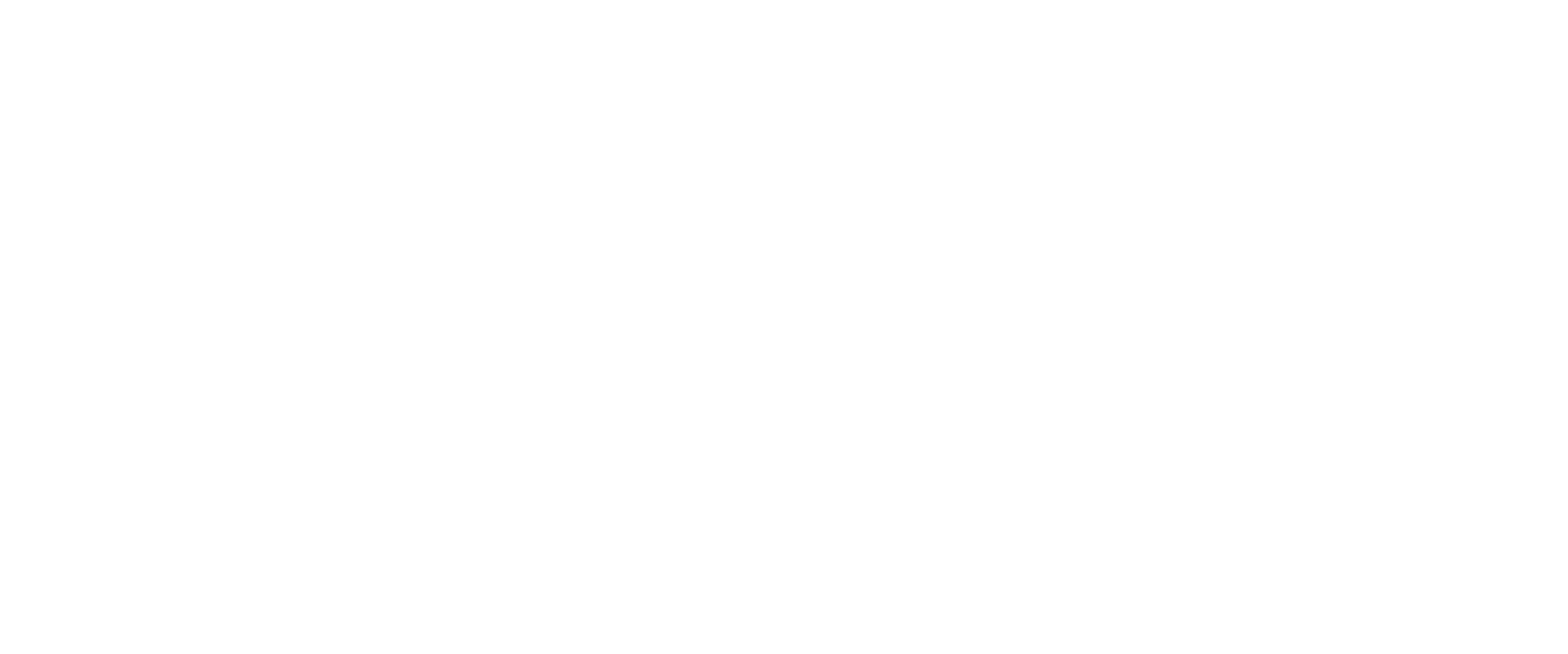# beuke.org

## A personal blog about computer science topics.

Applicative
A Strong Lax Monoidal Endofunctor
Posted on Sep 8 2023 ~ 7 min readAn applicative, in category theory, is a lax monoidal endofunctor with tensorial strength. Let $(\mathcal{C}, \otimes, 1_{\mathcal{C}})$ be a monoidal category. A lax monoidal endofunctor is a functor $F : \mathcal{C} \rightarrow \mathcal{C}$ together with two coherence maps:

• $\eta : 1_{\mathcal{C}} \rightarrow F(1_{\mathcal{C}})$ (the unit morphism)

• $\phi_{X,Y} : FX \otimes FY \rightarrow F(X \otimes Y)$ (a natural transformation)

such that the following diagrams commute:

\begin{xy} \xymatrix{ (FX\ \otimes\ FY)\ \otimes\ FZ \ar[r]^{\alpha} \ar[d]_{\phi_{X,Y}\ \otimes\ FZ} & FX\ \otimes\ (FY\ \otimes\ FZ) \ar[d]^{FX\ \otimes\ \phi_{Y,Z}} \\ F(X\ \otimes\ Y)\ \otimes\ FZ \ar[d]_{\phi_{X\ \otimes\ Y,Z}} & FX\ \otimes\ F(Y\ \otimes\ Z) \ar[d]^{\phi_{X,Y\ \otimes\ Z}} \\ F((X\ \otimes\ Y)\ \otimes\ Z) \ar[r]_{F_{\alpha}} & F(X\ \otimes\ (Y\ \otimes\ Z)) \\ } \end{xy}
\begin{xy} \xymatrix{ FX\ \otimes\ 1_{\mathcal{C}}\ar[d]_{\rho} \ar[r]^{FX\ \otimes\ \eta\ \ \ \ \ } & FX\ \otimes\ F(1_{\mathcal{C}}) \ar[d]^{\phi_{X,1_{\mathcal{C}}}} \\ FX & F(X\ \otimes\ 1_{\mathcal{C}}) \ar[l]_{F_{\rho}} } \end{xy}
\begin{xy} \xymatrix{ 1_{\mathcal{C}}\ \otimes\ FY\ar[d]_{\lambda} \ar[r]^{\eta\ \otimes\ FY\ \ \ \ } & F(1_{\mathcal{C}})\ \otimes\ F\ Y \ar[d]^{\phi_{1_{\mathcal{C},Y}}} \\ FY & F(1_{\mathcal{C}}\ \otimes\ Y) \ar[l]_{{F_{\lambda}}} } \end{xy}

The different natural transformations, $\alpha : (a \otimes b) \otimes c\ \rightarrow a \otimes (b \otimes c)$ (associativity), $\rho : a \otimes 1_{\mathcal{C}} \rightarrow a$ (right identity), $\lambda : 1_{\mathcal{C}} \otimes a \rightarrow a$ (left identity) are part of the monoidal structure on ${\mathcal {C}}$.

Applicative functors are a relatively new concept. They were first introduced in 2008 by Conor McBride and Ross Paterson in their paper Applicative programming with effects. In functional programming every functor is an endofunctor and every functor applied to the monoidal category $\mathbf{Set}$, with the tensor product replaced by cartesian product, inherently possesses a unique strength, resulting in every functor within $\mathbf{Set}$ being strong. In simpler terms, a strong lax monoidal functor is just a lax monoidal functor that also has the property of being a strong functor, and its strength coherently associates with the monoidal structure. When we apply this in the context of $\mathbf{Set}$ functors, this coherent association is automatically provided.

# Example

The Applicative typeclass in Haskell looks slightly different then our definition of a lax monidal functor. However there is another typeclass in Haskell called Monoidal that reflects our definition. Moreover, there is a equivalence between the two typeclasses Applicative and Monoidal. This parallels our previous demonstration of the interchangeability between bind and >>=, as discussed in my post on monads. Let me first introduce the typeclass Monoidal and then we show that this is equivalent to Applicative.

Please note that fa -> fb -> f(a, b) is actually the curried version of
(f a, f b) -> f (a, b)

Haskell comes with curry and uncurry as part of its standard library, which together form an isomorphism.

\begin{xy} \xymatrix { \texttt{(a, b) -> c} \ar@/^1.5pc/[rr]^{\texttt{curry}} && \texttt{a -> b -> c} \ar@/^1.5pc/[ll]^{\texttt{uncurry}}} \end{xy}

Hence we can also phrase Monoidal this way, and it aligns seamlessly with our categorical definition of a strong lax monoidal functor:

We have the usual monoidal laws (pseudocode):

Now that we have established the definition of Monoidal lets have a look at the equivalent Applicative definition in Haskell.

This is how to recover Applicative in terms of Monoidal:

And this is the reverse direction, Monoidal in terms of Applicative:

We’ve now formulated a two-way translation between Applicative and Monoidal, illustrating that they are isomorphic. This equality between Applicative and Monoidal can also be shown in a computer-checked proof in Coq. Now, lets have a look at some instances of Applicative.

An Instance of Applicative, the List Applicative

Another Instance, the Maybe Functor

All of the above is already implemented in the standard Haskell library, so you can also simply open an interactive Haskell interpreter (ghci) and test the following examples.

1. 0.The diagram displayed at the top of this post is a modified version of Brent Yorgey's Typeclassopedia diagram
2. 1.McBride, Conor; Paterson, Ross (2008-01-01). Applicative programming with effects (pdf). Journal of Functional Programming. 18 (1): 1–13. doi:10.1017/S0956796807006326
3. 2.Rivas, E., & Jaskelioff, M. (2014). Notions of Computation as Monoids. CoRR, abs/1406.4823. https://arxiv.org/abs/1406.4823